# 两地区人口流动肺结核动力学模型研究Dynamic Model of Tuberculosis in Population Migration between Two Regions

DOI: 10.12677/AAM.2019.83061, PDF, 下载: 402  浏览: 605  国家自然科学基金支持

Abstract: To study the effects of population migration and vaccination on the spread of tuberculosis, a dy-namic SEIR epidemic model of the two regions with different population densities is established. The effective reproduction number is calculated, and the vaccination strategy for each region is considered in model. We numerically simulate the sensitivity of the effective reproduction number with respect to migration rate and vaccination rate. The results show that migration from areas with high population density to areas with low population density can reduce the effective re-production number and disease scale. The migration from low-density areas to high-density areas has the opposite result, but they cannot achieve the purpose of eliminating tuberculosis through migration. Appropriate application of vaccines to people in the high-density area can eliminate tuberculosis.

1. 引言

2. 模型建立及再生数的计算

$\begin{array}{l}\frac{\text{d}{S}_{11}}{\text{d}t}={A}_{11}-{\sigma }_{12}{S}_{11}+{\rho }_{12}{S}_{12}-{S}_{11}\underset{j=1}{\overset{2}{\sum }}{k}_{1}{\beta }_{11j}\frac{{I}_{j1}}{{N}_{1}^{t}}-{\chi }_{11}{S}_{11}-d{S}_{11}\\ \frac{\text{d}{S}_{12}}{\text{d}t}={A}_{12}+{\sigma }_{12}{S}_{11}-{\rho }_{12}{S}_{12}-{S}_{12}\underset{j=1}{\overset{2}{\sum }}{k}_{2}{\beta }_{12j}\frac{{I}_{j2}}{{N}_{2}^{t}}-{\chi }_{12}{S}_{12}-d{S}_{12}\\ \frac{\text{d}{S}_{22}}{\text{d}t}={A}_{22}-{\sigma }_{21}{S}_{22}+{\rho }_{21}{S}_{21}-{S}_{22}\underset{j=1}{\overset{2}{\sum }}{k}_{1}{\beta }_{22j}\frac{{I}_{j2}}{{N}_{2}^{t}}-{\chi }_{22}{S}_{22}-d{S}_{22}\\ \frac{\text{d}{S}_{21}}{\text{d}t}={A}_{21}+{\sigma }_{21}{S}_{22}-{\rho }_{21}{S}_{21}-{S}_{21}\underset{j=1}{\overset{2}{\sum }}{k}_{1}{\beta }_{21j}\frac{{I}_{j1}}{{N}_{1}^{t}}-{\chi }_{21}{S}_{21}-d{S}_{21}\end{array}$

$\begin{array}{l}\frac{\text{d}{E}_{11}}{\text{d}t}={S}_{11}\underset{j=1}{\overset{2}{\sum }}{k}_{1}{\beta }_{11j}\frac{{I}_{j1}}{{N}_{1}^{t}}-{\sigma }_{12}{E}_{11}+{\rho }_{12}{E}_{12}-\left(\alpha +d\right){E}_{11}\\ \frac{\text{d}{E}_{12}}{\text{d}t}={S}_{12}\underset{j=1}{\overset{2}{\sum }}{k}_{2}{\beta }_{12j}\frac{{I}_{j2}}{{N}_{2}^{t}}+{\sigma }_{12}{E}_{11}-{\rho }_{12}{E}_{12}-\left(\alpha +d\right){E}_{12}\\ \frac{\text{d}{E}_{22}}{\text{d}t}={S}_{22}\underset{j=1}{\overset{2}{\sum }}{k}_{1}{\beta }_{22j}\frac{{I}_{j2}}{{N}_{2}^{t}}-{\sigma }_{21}{E}_{22}+{\rho }_{21}{E}_{21}-\left(\alpha +d\right){E}_{22}\\ \frac{\text{d}{E}_{21}}{\text{d}t}={S}_{21}\underset{j=1}{\overset{2}{\sum }}{k}_{1}{\beta }_{21j}\frac{{I}_{j1}}{{N}_{1}^{t}}+{\sigma }_{21}{E}_{22}-{\rho }_{21}{E}_{21}-\left(\alpha +d\right){E}_{21}\end{array}$

$\begin{array}{l}\frac{\text{d}{I}_{11}}{\text{d}t}=\alpha {E}_{11}-{\sigma }_{12}{I}_{11}+{\rho }_{12}{I}_{12}-\left(\gamma +d\right){I}_{11}\\ \frac{\text{d}{I}_{12}}{\text{d}t}=\alpha {E}_{12}+{\sigma }_{12}{I}_{11}-{\rho }_{12}{I}_{12}-\left(\gamma +d\right){I}_{12}\\ \frac{\text{d}{I}_{22}}{\text{d}t}=\alpha {E}_{22}-{\sigma }_{21}{I}_{22}+{\rho }_{21}{I}_{21}-\left(\gamma +d\right){I}_{22}\\ \frac{\text{d}{I}_{21}}{\text{d}t}=\alpha {E}_{21}+{\sigma }_{21}{I}_{22}-{\rho }_{21}{I}_{21}-\left(\gamma +d\right){I}_{21}\end{array}$

$\begin{array}{l}\frac{\text{d}{R}_{11}}{\text{d}t}=\gamma {I}_{11}-{\sigma }_{12}{R}_{11}+{\rho }_{12}{R}_{12}+{\chi }_{11}{S}_{11}-d{R}_{11}\\ \frac{\text{d}{R}_{12}}{\text{d}t}=\gamma {I}_{12}+{\sigma }_{12}{R}_{11}-{\rho }_{12}{R}_{12}+{\chi }_{12}{S}_{12}-d{R}_{12}\\ \frac{\text{d}{R}_{22}}{\text{d}t}=\gamma {I}_{22}-{\sigma }_{21}{R}_{22}+{\rho }_{21}{R}_{21}+{\chi }_{22}{S}_{22}-d{R}_{22}\\ \frac{\text{d}{R}_{21}}{\text{d}t}=\gamma {I}_{21}+{\sigma }_{21}{R}_{22}-{\rho }_{21}{R}_{21}+{\chi }_{21}{S}_{21}-d{R}_{21}\end{array}$ (1)

$\begin{array}{l}{N}_{1}^{t}={S}_{11}\left(t\right)+{S}_{21}\left(t\right)+{E}_{11}\left(t\right)+{E}_{21}\left(t\right)+{I}_{11}\left(t\right)+{I}_{21}\left(t\right)+{R}_{11}\left(t\right)+{R}_{21}\left(t\right)\\ {N}_{2}^{t}={S}_{12}\left(t\right)+{S}_{22}\left(t\right)+{E}_{12}\left(t\right)+{E}_{22}\left(t\right)+{I}_{12}\left(t\right)+{I}_{22}\left(t\right)+{R}_{12}\left(t\right)+{R}_{22}\left(t\right)\end{array}$Table 1. The meaning of parameter in model

$\begin{array}{l}\frac{\text{d}{S}_{11}}{\text{d}t}={A}_{11}-{\sigma }_{12}{S}_{11}+{\rho }_{12}{S}_{12}-d{S}_{11}\\ \frac{\text{d}{S}_{12}}{\text{d}t}={A}_{12}+{\sigma }_{12}{S}_{11}-{\rho }_{12}{S}_{12}-d{S}_{12}\\ \frac{\text{d}{S}_{22}}{\text{d}t}={A}_{22}-{\sigma }_{21}{S}_{22}+{\rho }_{21}{S}_{21}-d{S}_{22}\\ \frac{\text{d}{S}_{21}}{\text{d}t}={A}_{21}+{\sigma }_{21}{S}_{22}-{\rho }_{21}{S}_{21}-d{S}_{21}\end{array}$ (2)

${S}_{011}^{*}=\frac{{\rho }_{12}\left({A}_{11}+{A}_{12}\right)+{A}_{11}d}{\left({\rho }_{12}+d\right)\left({\sigma }_{12}+d\right)-{\sigma }_{12}{\rho }_{12}},{S}_{012}^{*}=\frac{{A}_{12}d+{\sigma }_{12}\left({A}_{11}+{A}_{12}\right)}{\left({\rho }_{12}+d\right)\left({\sigma }_{12}+d\right)-{\sigma }_{12}{\rho }_{12}}$

${S}_{022}^{*}=\frac{{\rho }_{21}\left({A}_{21}+{A}_{22}\right)+{A}_{22}d}{\left({\rho }_{21}+d\right)\left({\sigma }_{21}+d\right)-{\sigma }_{21}{\rho }_{21}},{S}_{021}^{*}=\frac{{A}_{21}d+{\sigma }_{21}\left({A}_{21}+{A}_{22}\right)}{\left({\rho }_{21}+d\right)\left({\sigma }_{21}+d\right)-{\sigma }_{21}{\rho }_{21}}$

$\left(\begin{array}{cccc}-{\sigma }_{12}-d& {\rho }_{12}& 0& 0\\ {\sigma }_{12}& -{\rho }_{12}-d& 0& 0\\ 0& 0& -{\sigma }_{21}-d& {\rho }_{21}\\ 0& 0& {\sigma }_{21}& -{\rho }_{21}-d\end{array}\right)$

$\begin{array}{l}\frac{\text{d}{S}_{11}}{\text{d}t}={A}_{11}-{\sigma }_{12}{S}_{11}+{\rho }_{12}{S}_{12}-{\chi }_{11}{S}_{11}-d{S}_{11}\\ \frac{\text{d}{S}_{12}}{\text{d}t}={A}_{12}+{\sigma }_{12}{S}_{11}-{\rho }_{12}{S}_{12}-{\chi }_{12}{S}_{12}-d{S}_{12}\\ \frac{\text{d}{S}_{22}}{\text{d}t}={A}_{22}-{\sigma }_{21}{S}_{22}+{\rho }_{21}{S}_{21}-{\chi }_{22}{S}_{22}-d{S}_{22}\\ \frac{\text{d}{S}_{21}}{\text{d}t}={A}_{21}+{\sigma }_{21}{S}_{22}-{\rho }_{21}{S}_{21}-{\chi }_{21}{S}_{21}-d{S}_{21}\end{array}$

$\begin{array}{l}\frac{\text{d}{R}_{11}}{\text{d}t}={\sigma }_{12}{R}_{11}+{\rho }_{12}{R}_{12}+{\chi }_{11}{S}_{11}-d{R}_{11}\\ \frac{\text{d}{R}_{12}}{\text{d}t}={\sigma }_{12}{R}_{11}-{\rho }_{12}{R}_{12}+{\chi }_{12}{S}_{12}-d{R}_{12}\\ \frac{\text{d}{R}_{22}}{\text{d}t}={\sigma }_{21}{R}_{22}+{\rho }_{21}{R}_{21}+{\chi }_{22}{S}_{22}-d{R}_{22}\\ \frac{\text{d}{R}_{21}}{\text{d}t}={\sigma }_{21}{R}_{22}-{\rho }_{21}{R}_{21}+{\chi }_{21}{S}_{21}-d{R}_{21}\end{array}$ (3)

${S}_{111}^{*}=\frac{{A}_{11}\left({\chi }_{12}+d\right)+{\rho }_{12}\left({A}_{11}+{A}_{12}\right)}{\left({\rho }_{12}+{\chi }_{12}+d\right)\left({\sigma }_{12}+{\chi }_{11}+d\right)-{\sigma }_{12}{\rho }_{12}},{S}_{112}^{*}=\frac{{A}_{12}\left({\chi }_{11}+d\right)+{\sigma }_{12}\left({A}_{11}+{A}_{12}\right)}{\left({\rho }_{12}+{\chi }_{12}+d\right)\left({\sigma }_{12}+{\chi }_{11}+d\right)-{\sigma }_{12}{\rho }_{12}}$

${S}_{122}^{*}=\frac{{A}_{22}\left({\chi }_{21}+d\right)+{\rho }_{21}\left({A}_{22}+{A}_{21}\right)}{\left({\rho }_{21}+{\chi }_{21}+d\right)\left({\sigma }_{21}+{\chi }_{22}+d\right)-{\sigma }_{21}{\rho }_{21}},{S}_{121}^{*}=\frac{{A}_{21}\left({\chi }_{22}+d\right)+{\sigma }_{21}\left({A}_{22}+{A}_{21}\right)}{\left({\rho }_{21}+{\chi }_{21}+d\right)\left({\sigma }_{21}+{\chi }_{22}+d\right)-{\sigma }_{21}{\rho }_{21}}$

${R}_{111}^{*}=\frac{{\rho }_{12}{\chi }_{12}{S}_{112}^{*}+\left({\rho }_{12}+d\right){\chi }_{11}{S}_{111}^{*}}{d\left({\sigma }_{12}+{\rho }_{12}+d\right)},{R}_{112}^{*}=\frac{{\sigma }_{12}{\chi }_{11}{S}_{111}^{*}+\left({\sigma }_{12}+d\right){\chi }_{12}{S}_{112}^{*}}{d\left({\sigma }_{12}+{\rho }_{12}+d\right)}$

${R}_{122}^{*}=\frac{{\rho }_{21}{\chi }_{21}{S}_{121}^{*}+\left({\rho }_{21}+d\right){\chi }_{22}{S}_{22}^{*}}{d\left({\sigma }_{21}+{\rho }_{21}+d\right)},{R}_{121}^{*}=\frac{{\sigma }_{21}{\chi }_{22}{S}_{122}^{*}+\left({\sigma }_{21}+d\right){\chi }_{21}{S}_{121}^{*}}{d\left({\sigma }_{21}+{\rho }_{21}+d\right)}$

${N}_{1}^{t*}={S}_{111}^{*}+{S}_{121}^{*}\text{+}{R}_{111}^{*}+{R}_{121}^{*},{N}_{2}^{t*}={S}_{222}^{*}+{S}_{212}^{*}+{R}_{222}^{*}+{R}_{212}^{*}$

$\mathcal{F}=\left(\begin{array}{c}{S}_{11}\underset{j=1}{\overset{2}{\sum }}{k}_{1}{\beta }_{11j}\frac{{I}_{j1}}{{N}_{1}^{t}}\\ {S}_{12}\underset{j=1}{\overset{2}{\sum }}{k}_{2}{\beta }_{12j}\frac{{I}_{j2}}{{N}_{2}^{t}}\\ {S}_{22}\underset{j=1}{\overset{2}{\sum }}{k}_{1}{\beta }_{22j}\frac{{I}_{j2}}{{N}_{2}^{t}}\\ {S}_{21}\underset{j=1}{\overset{2}{\sum }}{k}_{1}{\beta }_{21j}\frac{{I}_{j1}}{{N}_{1}^{t}}\\ 0\\ 0\\ 0\\ 0\end{array}\right)$ , $\mathcal{V}=\left(\begin{array}{c}{\sigma }_{12}{E}_{11}-{\rho }_{12}{E}_{12}+\left(\alpha +d\right){E}_{11}\\ -{\sigma }_{12}{E}_{11}+{\rho }_{12}{E}_{12}+\left(\alpha +d\right){E}_{12}\\ {\sigma }_{21}{E}_{22}-{\rho }_{21}{E}_{21}+\left(\alpha +d\right){E}_{22}\\ -{\sigma }_{21}{E}_{22}+{\rho }_{21}{E}_{21}+\left(\alpha +d\right){E}_{21}\\ -\alpha {E}_{11}+{\sigma }_{12}{I}_{11}-{\rho }_{12}{I}_{12}+\left(\gamma +d\right){I}_{11}\\ -\alpha {E}_{12}-{\sigma }_{12}{I}_{11}+{\rho }_{12}{I}_{12}+\left(\gamma +d\right){I}_{12}\\ -\alpha {E}_{22}+{\sigma }_{21}{I}_{22}-{\rho }_{21}{I}_{21}+\left(\gamma +d\right){I}_{22}\\ -\alpha {E}_{21}-{\sigma }_{21}{I}_{22}+{\rho }_{21}{I}_{21}+\left(\gamma +d\right){I}_{21}\end{array}\right)$

$\begin{array}{l}{m}_{12}={\sigma }_{12}+\alpha +d,{n}_{12}={\rho }_{12}+\alpha +d,{m}_{21}={\sigma }_{21}+\alpha +d,{n}_{21}={\rho }_{21}+\alpha +d,\\ {p}_{12}={\sigma }_{12}+r+d,{q}_{12}={\rho }_{12}+r+d,{p}_{21}={\sigma }_{21}+r+d,{q}_{21}={\rho }_{21}+r+d\end{array}$

$\mathcal{F},\mathcal{V}$ 分别关于潜伏项 ${E}_{ij}$ 与染病项 ${I}_{ij}$ 求导得 $F,V$

$F=\left(\begin{array}{cc}0& {F}_{12}\\ 0& 0\end{array}\right)$ ，其中 ${F}_{12}=\left(\begin{array}{cccc}\frac{{S}_{11}^{*}{k}_{1}{\beta }_{111}}{{N}_{1}^{t*}}& 0& 0& \frac{{S}_{11}^{*}{k}_{1}{\beta }_{112}}{{N}_{1}^{t*}}\\ 0& \frac{{S}_{12}^{*}{k}_{2}{\beta }_{121}}{{N}_{2}^{t*}}& \frac{{S}_{12}^{*}{k}_{2}{\beta }_{122}}{{N}_{2}^{t*}}& 0\\ 0& \frac{{S}_{22}^{*}{k}_{2}{\beta }_{221}}{{N}_{2}^{t*}}& \frac{{S}_{22}^{*}{k}_{2}{\beta }_{222}}{{N}_{2}^{t*}}& 0\\ \frac{{S}_{21}^{*}{k}_{1}{\beta }_{211}}{{N}_{1}^{t*}}& 0& 0& \frac{{S}_{21}^{*}{k}_{1}{\beta }_{212}}{{N}_{1}^{t*}}\end{array}\right)$

$V=\left(\begin{array}{cc}{V}_{11}& 0\\ {V}_{21}& {V}_{22}\end{array}\right)$ ，其中 

${V}_{21}=\left(\begin{array}{cccc}-\alpha & 0& 0& 0\\ 0& -\alpha & 0& 0\\ 0& 0& -\alpha & 0\\ 0& 0& 0& -\alpha \end{array}\right)$ , ${V}_{22}=\left(\begin{array}{cccc}{p}_{12}& -{\rho }_{12}& 0& 0\\ -{\sigma }_{12}& {q}_{12}& 0& 0\\ 0& 0& {p}_{21}& -{\rho }_{21}\\ 0& 0& -{\sigma }_{21}& {q}_{21}\end{array}\right)$

${V}_{11}^{-1}=\left(\begin{array}{cccc}\frac{{n}_{12}}{{m}_{12}{n}_{12}-{\rho }_{12}{\sigma }_{12}}& \frac{{\rho }_{12}}{{m}_{12}{n}_{12}-{\rho }_{12}{\sigma }_{12}}& 0& 0\\ \frac{{\sigma }_{12}}{{m}_{12}{n}_{12}-{\rho }_{12}{\sigma }_{12}}& \frac{{m}_{12}}{{m}_{12}{n}_{12}-{\rho }_{12}{\sigma }_{12}}& 0& 0\\ 0& 0& \frac{{n}_{21}}{{m}_{21}{n}_{21}-{\rho }_{21}{\sigma }_{21}}& \frac{{\rho }_{21}}{{m}_{21}{n}_{21}-{\rho }_{21}{\sigma }_{21}}\\ 0& 0& \frac{{\sigma }_{21}}{{m}_{21}{n}_{21}-{\rho }_{21}{\sigma }_{21}}& \frac{{m}_{12}}{{m}_{21}{n}_{21}-{\rho }_{21}{\sigma }_{21}}\end{array}\right)$

${V}_{22}^{-1}=\left(\begin{array}{cccc}\frac{{q}_{12}}{{p}_{12}{q}_{12}-{\rho }_{12}{\sigma }_{12}}& \frac{{\rho }_{12}}{{p}_{12}{q}_{12}-{\rho }_{12}{\sigma }_{12}}& 0& 0\\ \frac{{\sigma }_{12}}{{p}_{12}{q}_{12}-{\rho }_{12}{\sigma }_{12}}& \frac{{p}_{12}}{{p}_{12}{q}_{12}-{\rho }_{12}{\sigma }_{12}}& 0& 0\\ 0& 0& \frac{{q}_{21}}{{p}_{21}{q}_{21}-{\rho }_{21}{\sigma }_{21}}& \frac{{\rho }_{21}}{{p}_{21}{n}_{21}-{\rho }_{21}{\sigma }_{21}}\\ 0& 0& \frac{{\sigma }_{21}}{{p}_{21}{q}_{21}-{\rho }_{21}{\sigma }_{21}}& \frac{{p}_{21}}{{p}_{21}{q}_{21}-{\rho }_{21}{\sigma }_{21}}\end{array}\right)$

${U}_{12}={m}_{12}{n}_{12}-{\rho }_{12}{\sigma }_{12},{U}_{21}={m}_{21}{n}_{21}-{\rho }_{21}{\sigma }_{21},{W}_{12}={p}_{12}{q}_{12}-{\rho }_{12}{\sigma }_{12},{W}_{21}={p}_{21}{q}_{21}-{\rho }_{21}{\sigma }_{21}$ ，有

$-{V}_{22}^{-1}{V}_{21}{V}_{11}^{-1}=\left(\begin{array}{cccc}\frac{\alpha \left({n}_{12}{q}_{12}+{\sigma }_{12}{\rho }_{12}\right)}{{W}_{12}{U}_{12}}& \frac{\alpha \left({\rho }_{12}{q}_{12}+{m}_{12}{\rho }_{12}\right)}{{W}_{12}{U}_{12}}& 0& 0\\ \frac{\alpha \left({\sigma }_{12}{\eta }_{12}+{\sigma }_{12}{p}_{12}\right)}{{W}_{12}{U}_{12}}& \frac{\alpha \left({\sigma }_{12}{\rho }_{12}+{p}_{12}{m}_{12}\right)}{{W}_{12}{U}_{12}}& 0& 0\\ 0& 0& \frac{\alpha \left({n}_{21}{q}_{21}+{\sigma }_{21}{\rho }_{21}\right)}{{W}_{21}{U}_{21}}& \frac{\alpha \left({\rho }_{21}{q}_{21}+{m}_{21}{\rho }_{21}\right)}{{W}_{21}{U}_{21}}\\ 0& 0& \frac{\alpha \left({\sigma }_{21}{\eta }_{21}+{\sigma }_{21}{p}_{21}\right)}{{W}_{21}{U}_{21}}& \frac{\alpha \left({\sigma }_{21}{\rho }_{21}+{p}_{21}{m}_{21}\right)}{{W}_{21}{U}_{12}}\end{array}\right)$

${K}_{11}=-{F}_{12}{V}_{22}^{-1}{V}_{21}{V}_{11}^{-1}$ ，则有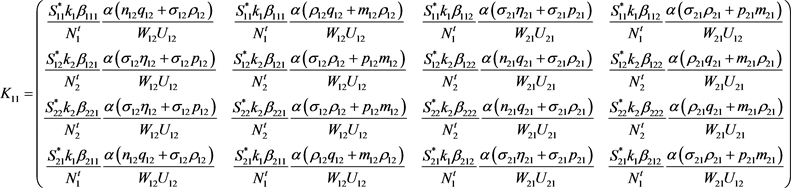3. 数值模拟Table 2. The value of parameter in simulation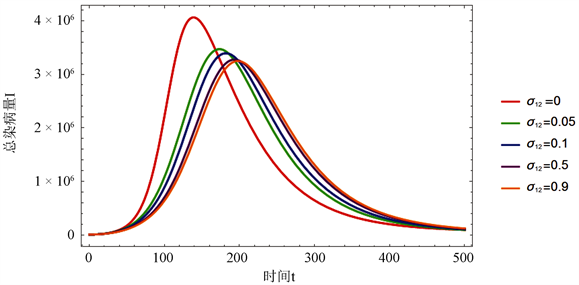Figure 1. Trend graph of total infected I with time at different migration rates ${\sigma }_{12}$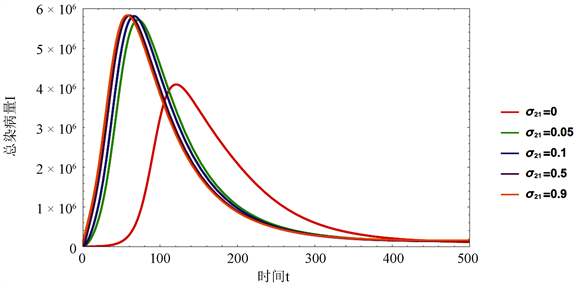Figure 2. Trend graph of total infected I with time at different migration rates ${\sigma }_{21}$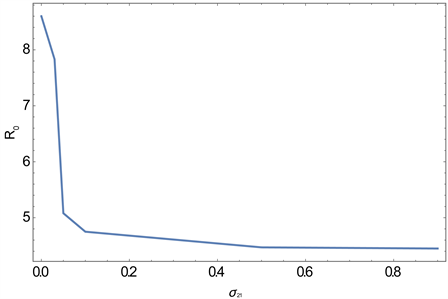Figure 3. Graph of relation between ${\sigma }_{12}$ and ${R}_{0}$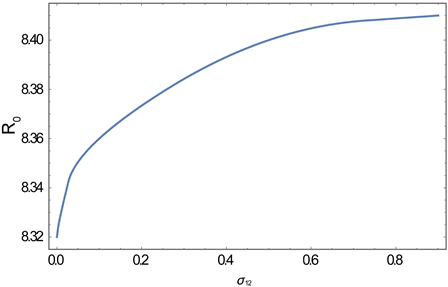Figure 4. Graph of relation between ${\sigma }_{21}$ and ${R}_{0}$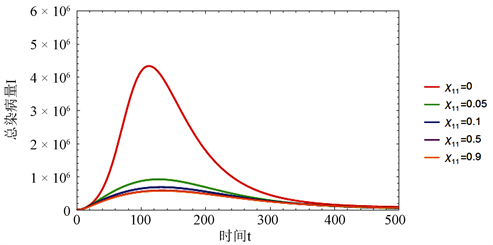Figure 5. Graph of of relation between ${\chi }_{11}$ and total infected IFigure 6. Graph of relation between ${\chi }_{11}$ and ${R}_{v}$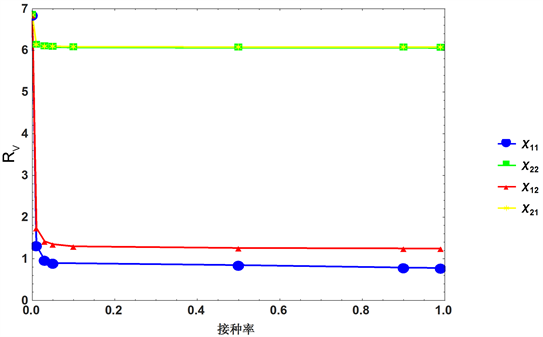Figure 7. Graph of relationship between vaccine rate in each group and ${R}_{v}$

4. 结论

  陈启军, 陈越, 杜生明. 论传染病的危害及我国的防治策略[J]. 中国基础科学, 2005, 7(6): 21-32.  张兰. 关于公共卫生传染病控制的探索[J]. 大家健康(学术版), 2014(20): 29-30.  张金慧. 肺结核传播模型的定性分析及数据模拟[D]: [博士学位论文]. 武汉: 华中师范大学, 2014.  Xu, C., Wei, X., Cui, J., et al. (2017) Mixing in Regional-Structure Model about the Influence of Floating Population and Optimal Control about TB in Guangdong Province of China. International Journal of Biomathematics, 10, 12. https://doi.org/10.1142/S1793524517501066  Zhou, Y., Khan, K., Feng, Z., et al. (2008) Projection of Tu-berculosis Incidence with Increasing Immigration Trends. Journal of Theoretical Biology, 254, 215-228. https://doi.org/10.1016/j.jtbi.2008.05.026  朱宏淼, 靳祯. 两个城市人口相互流动的流感模型研究[J]. 数学的实践与认识, 2012, 42(6): 103-110.  国家数据. http://data.stats.gov.cn/easyquery.htm?cn=C01  van den Driessche, P. and Watmough, J. (2002) Reproduction Numbers and Sub-Threshold Endemic Equilibria for Com-partmental Models of Disease Transmission. Mathematical Biosciences, 180, 29-48. https://doi.org/10.1016/S0025-5564(02)00108-6  Feng, Z, Hill, A.N., Curns, A.T., et al. (2016) Evaluating Targeted Interventions via Meta-Population Models with Multi-Level Mixing. Mathematical Biosciences, 287, 93-104. https://doi.org/10.1016/j.mbs.2016.09.013